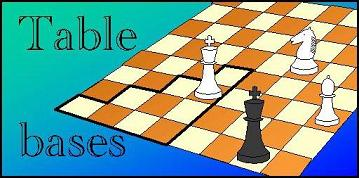Leapfrog: Symmetry# Symmetry of Chess Positions: Theory

### Pawnless Chess has 8-fold symmetry

A square Chess board has 8-fold symmetry, i.e. it can be put in 8 different orientations on top of itself, without it making any difference (we neglect the coloring of the squares, which has no effect on the game). All pieces except Pawns also have this symmetry in their moves, so Pawn-less positions which result from rotating or reflecting the board are really equivalent. The eight symmetry operations can all be made from just three basic elements: horizontal reflection (mapping the 1st rank on the 8th rank), vertical reflection (mapping the a-file on the h-file), and diagonal reflection (mapping the a-file on the 1st rank). E.g. a horizontal reflection, followed by a vertical one, gives a 180-degree rotation. Note that the order is important: diagonal reflection after horizontal reflection gives a 90-degree counter-clockwise rotation, while doing the diagonal reflection first would make it a clockwise rotation.

We will indicate the three basic reflections by D, H and V. Note that when we do the same reflection twice, they cancel each other. Note that H and V commute, i.e. it does not matter in which order you apply them. If we write HV for the combined operation of first doing H and then V, we have HV = VH. For D we already saw that this is not true: a diagonal reflection swaps the horizontal for the vertical. So doing a horizontal reflection before it, is the same as doing a vertical reflection after it. I.e. DH = VD and DV = HD.

With this knowledge, we can write every symmetry operation as a combination of H, V and D, in that order (but not necessarily all present). There never needs to be more than one of each, as you can always swap the order (converting H to V and vice versa, if needed) to get identical operations immediately next to each other, where they cancel. E.g. HVDHDVHD = HV(DH)DVHD = HV(VD)DVHD = H(VV)(DD)VHD = HVHD = (HV)HD = (VH)HD = V(HH)D = VD. (The parentheses indicate which operations we were swapping the order of, or were cancelling.) There are thus only 8 symmetry operations, characterized by H, V and D being present or not, namely H, V, D, HV (= 180-deg rotation), HD (90-deg counter-clockwise rotation), VD (90-deg clockwise rotation), HVD (reflection about the 'anti-diagonal' a8-h1), and of course the identity operation which leaves the board in its original orientation.

Note that for non-square boards, diagonal reflection is not a symmetry operation, and we will have only 4-fold symmetry. The presence of Pawns would break the symmetry w.r.t. horizontal reflection. Positions with Pawns can still have symmetry w.r.t. vertical reflection, (2-fold symmetry) when the Pawns are symmetrically positioned in pairs.

### Canonical Representative

Positions that are images of each other under some symmetry transformation, are completely equivalent. Thi means they will have the same winnin-distance, brought about by the (similarly transformed) same sequence of moves. There is no need to spend any time on such a position, if we have already solved an equivalent one. So in general, each 8 possible orientations and reflections of a position will be represented in our EGT by only one member of the group. We will call this the canonica representative.

When all pieces are different, the canonical representative is usually defined a the position that has the designated primary symmetry piece in the triangle a1-d1-d4. If this primary piece is on the diagonal a1-d4, a designated secondary piece has to be in the triangle a1-a8-h8.

This is not enough to weed out all symmetry images: if both primary and secondary symmetry piece are on the diagonal, there are still two positions, differing by the location of the other pieces being each other's diagonal image, which satisfy our definition of 'canonical reprsentative'. Since their number is only a very small fraction of the total number of positions, and it would be a hassle to start paying attention to a teriary symmetry piece, we take this overhead for granted.

### Exchange symmetry

When two of the pieces are of equal type, exchanging their location also does not produce an observable difference. The leapfrog generator only uses this for reducing the EGT size if the two identical pieces are white. In that case it uses these two pieces as primary and secondary symmetry piece. This means that the definition of 'canonical representative' has to be extended, to indicate which version of the exchange images is canonical, and which not. We now require the middle of the line connecting the symmetry pieces to fall in the triangle limited by the board diagonal, the 1st-rank board edge, and the line between the d- and e-file.

Because the center of the line connecting two pieces can be on a grid of half-squares, we now not only have to add further conditions to resolve the cases where this center is on the diagonal, but also when it is on the vertical board axis. We require that the position will be reflected around D or V such that the piece closest to the board center is below the diagonal (for D) or on the left half of the board (for V). As for exchangng the pieces, we require that the first piece is on the square with the lowest number.